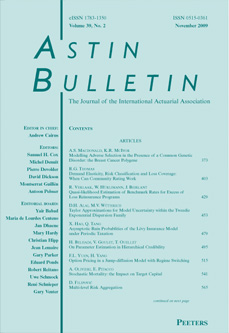this issueprevious article in this issue next article in this issueDocument Details : Title: Pseudo Compound Poisson Distributions in Risk Theory Author(s): HÜRLIMANN, W. Journal: ASTIN Bulletin Volume: 20    Issue: 1   Date: April 1990   Pages: 57-79 DOI: 10.2143/AST.20.1.2005483 Abstract : Using Laplace transforms and the notion of a pseudo compound Poisson distribution, some risk theoretical results are revisited A well-known theorem by FELLER (1968) and VAN HARN (1978) on infinitely divisible distributions is generalized. The result may be used for the efficient evaluation of convolutions for some distributions. In the particular arithmetic case, alternate formulae to those recently proposed by DE PRIL (1985) are derived and shown more adequate in some cases. The individual model of risk theory is shown to be pseudo compound Poisson. It is thus computable using numerical tools from the theory of integral equations m the continuous case, a formula of Panjer type or the Fast Fourier transform in the arithmetic case. In particular our results contain some of DE PRIL'S (1986/89) recursive formulae for the individual life model with one and multiple causes of decrement. As practical illustration of the continuous case we construct a new two-parametric family of claim size density functions whose corresponding compound Poisson distributions are analytical finite sum expressions. Analytical expressions for the finite and infinite time ruin probabilities are also derived.

44.210.21.70.